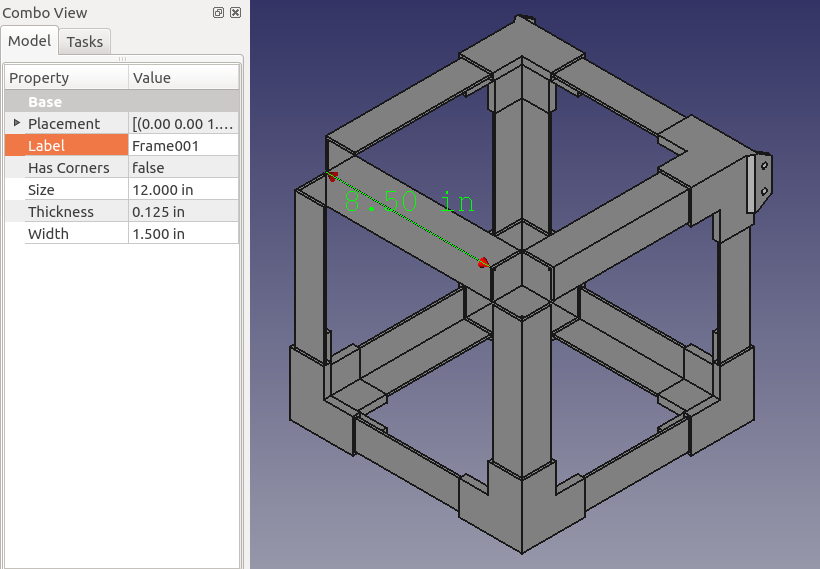# Generate Cut List¶

There are two options in the main menu to generate a cut list:

1.Copy Cut List to Clipboard

2.Save Cut List as CSV

Both options generate a cut list with the following:

• Rods for axes, heated bed, and spool holder

• Angled bars to construct a frame with 3d printable corners

## How it Works¶

Each option queries the active document for Axis objects and the Frame object in order to determine the rods and angled bars for the cut list.

### Determining Rod Quantity¶

• 2 X Axis Rods are added for every X Axis object

• 2 Y Axis Rods are added or every Y Axis object

• 2 Z Axis Rods are added for every Z Axis object

• 3 Spool Holder Rods are added based on the existence of a Frame object

• 2 Heated Bed Rods are added for every pair of Z Axis objects

### Determining Rod Length¶

• Y Axis Rod Length corresponds with `Length` of the axis in the document

• Z Axis Rod Length is adjusted by subtracting 1 inch

• Length of Heated Bed Rods and 1 Spool Holder Rod are equal to the length of the Frame

• Length of 2 Spool Holder Rods are equal to the length of the Frame minus 1 inch (similar to Z axis rods)

### Determining Angled Bar Quantity¶

• 12 Angled Bars are added based on the existence of a Frame object

### Determining Angled Bar Length¶

Angled bar length is calculated from the following formula:

```Frame.Size - ((Frame.Width + (Frame.Thickness * 2)) * 2)
```

For example, a 12 in frame with a 1.5 in `Width` and 0.125 in `Thickness` could have 8.5 in angled bars.

```12 in - ((1.5 in + (0.125 in * 2)) * 2) = 8.5 in
```